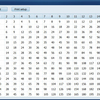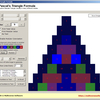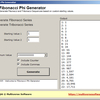# Mathematics (archive 28)

Mathematics software## Times Table

Create and print custom time tables of different values and sizes.## Pascal's Triangle Formula

Explore Pascal's Triangle visually. Pascal's Triangle Formula lets you zoom in and modify many properties of the triangle in a visual way. Pascal's Triangle is a famous and simple ...## Fibonacci Phi Generator

Generate custom Fibonnaci and Tribonacci sequences with this small mathematics tool. Select your own custom starting numbers. These numbers will be used to generate these special m ...## Pascal's Triangle Formula

Explore Pascal's Triangle visually. Pascal's Triangle Formula lets you zoom in and modify many properties of the triangle in a visual way. Pascal's Triangle is a famous and simple ...